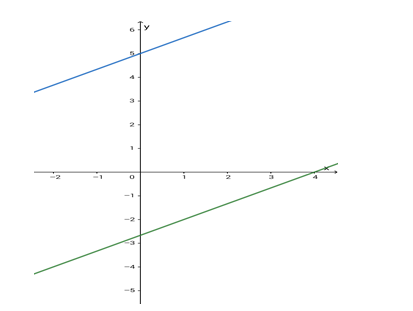# Find the equation of the line which is parallel to the line

Question:

Find the equation of the line which is parallel to the line 2x – 3y = 8 and whose y - intercept is 5 units.

Solution:Given: The given line is $2 x-3 y=8$. The line parallel to this line has a $y$-intercept of 5 units.

Formula to be used: If $a x+b y=c$ is a straight line then the line parallel to the given line is of the form $a x+b y=d$, where $a, b, c, d$ are arbitrary real constants.

A line parallel to the given line has a slope of $\frac{2}{3}$ and is of the form $2 x-3 y=k$, where $k$ is any arbitrary real constant.

Now, $2 x-3 y=k$

or, $3 y=2 x-k$

or, $y=\left(\frac{2}{3}\right) x+\left(-\frac{k}{3}\right)$

which is of the form y = mx + c , where c is the y - intercept.

$\therefore \mathrm{c}=-\frac{\mathrm{k}}{3}=5$

So, $k=(-3) \times 5=-15$

Equation of the required line is 2x - 3y = - 15

i.e. $2 x-3 y+15=0$